## Example Questions

### Example Question #1 : Number Line

The range of the earnings for architecture graduates is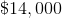, and the range of the salaries for engineering graduates is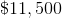.

Which of the following statements individually provide(s) sufficient additional information to determine the range of the salaries of all graduates between the two professions?

A: The median salary for the engineers is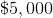greater than that of the architects.

B: The average (arithmetic mean) of the engineers is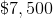greater than that of the architects.

C: The lowest salary of the engineers is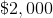less than the lowest of the architects.

B only

C only

A only

A, B, and C

A and C only

C only

Explanation:

The provision of the bottom-end of the engineering range is the only additional information that provides us a fixed endpoint from which we can build off of by supplementing with the ranges provided in the question, to give us the full range between both engineering and achitecture graduates. See the diagram provided to understand how this can be done.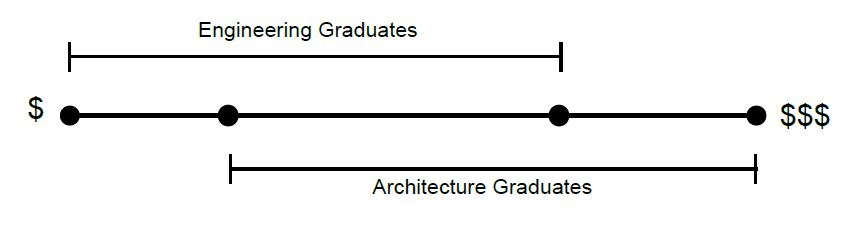Even if the mean and medians were provided, these additional values give us no information on the endpoints of the salaries, and the question only asks for the range.

### Example Question #2 : Number Line

What's the distance betweenandon a number line?Explanation:

Let's draw a number line.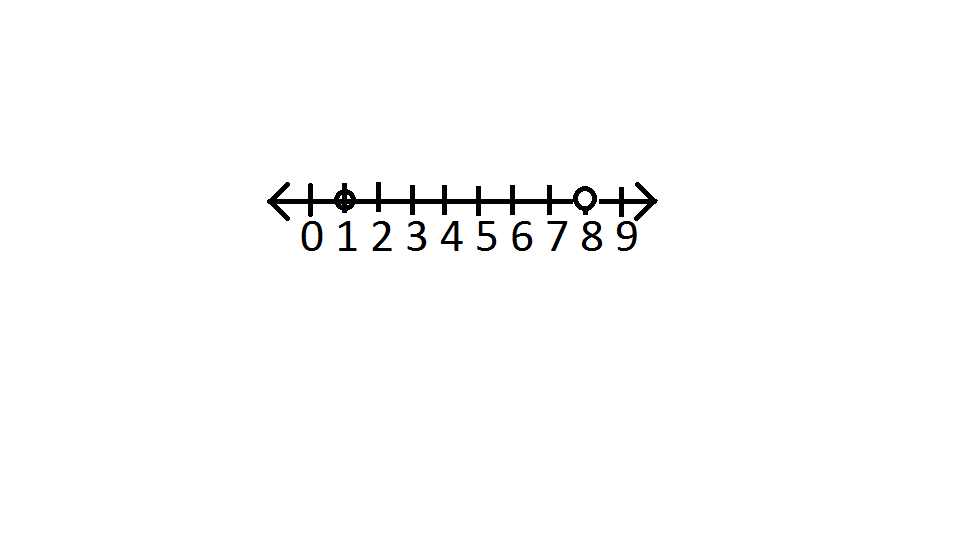Since a number line is straight and contains the numbers consecutively, we just subtractfromto get### Example Question #3 : Number Line

Which of the following answer best fits in the picture below?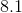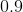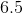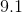Explanation:

Open circles mean the values are excluded from the set.

The number line shows the set is betweenandexclusive.

The only value in that set would be### Example Question #4 : Number Line

If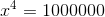, then where on the number line lies?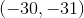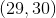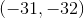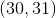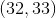Explanation:

Because a number line contains both positive and negative integers, we need to consider both possibilities.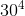is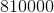and that value is the same as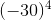. Therefore we eliminate the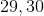choice because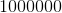will always be greater than those values raised to the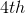power.

Next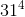is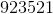. We elminate both the positive and negative range of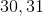. If we look at the difference betweenand, it's over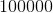.

Then, we should guess that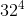will definitely be greater thanso therefore answer is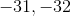.

Remember, a negative value raised to an even power will always have a positive value.

### Example Question #5 : Number Line

If perimeter of equilateral triangle is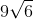, what is the height of the triangle?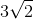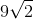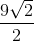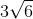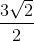Explanation:

Since perimeter of equilateral triangle isand we have three equal sides, we just divide that vaue byto get. To find height, we can set-up a proportion.

The height is opposite the angle. Side oppositeis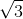and the side of equilateral triangle which is oppositeis.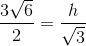Cross multiply.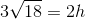Divide both sides by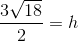Let's simplify by factoring out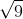to get a final answer ofTired of practice problems?

Try live online GRE prep today.